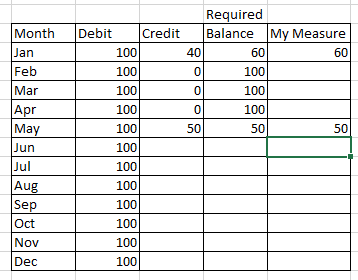cancel
Showing results for
Did you mean:Helper I

## Dax Measure

I need help with a measure to get result as shown below in column Balance.  If there is no value in Credit column then I dont want to show YTD balance. The measure that I have is

IF(Table1[Credit]=BLANK(),BLANK(),Table1[Debit]-Table2[Credit])

which is giving me blanks where the value is 0.1 ACCEPTED SOLUTIONCommunity Support

HI @NishPatel,

You can try to use the following measure formula if it suitable for your requirement:

``````VarianceMeasure =
VAR currDate =
MAX ( DebitTable[Date] )
RETURN
IF (
[CreditMeasure] = BLANK (),
SUMX (
SUMMARIZE (
FILTER ( ALLSELECTED ( DebitTable ), [Date] <= currDate ),
[Month],
[Date],
"Debit", SUM ( DebitTable[Amount] ),
"Credit",
CALCULATE (
SUM ( CreditTable[Amount] ),
FILTER (
ALLSELECTED ( CreditTable ),
CreditTable[Date] IN VALUES ( DebitTable[Date] )
)
)
),
[Debit] - [Credit]
),
[DebitMeasure] - [CreditMeasure]
)``````

Regards,

Xiaoxin Sheng

Community Support Team _ Xiaoxin
If this post helps, please consider accept as solution to help other members find it more quickly.
3 REPLIES 3Resident Rockstar

I think the general problem is that you work with two different tables and want to build a measure without aggregation functions.

Can you please share your data model with a picture as well as the structure of table 1 and table 2. Do you use an additional date table?

Best regards

Michael

------------------------------------------------------------------
Visit my blog datenhungrig which I recently started with content about business intelligence and Power BI in German and English or follow me on LinkedIn!Helper I

Hi Mike, First of all thank you for taking your time to help me out here. Because of the confidentiality of the data, I am unable to share my original table/pbix file. But below are the sample data tables that I have created which are almost similar to my data. Month Table is join by month with other two tables. And I have below 3 measure as well.DebitMeasure = CALCULATE(SUM(DebitTable[Amount]),SUMMARIZE(DebitTable, DebitTable [Month]))

CreditMeasure = CALCULATE(SUM(CreditTable[Amount]),SUMMARIZE(CreditTable, DebitTable [Month]))

VarianceMeasure =  IF(CreditTable[CreditMeasure]=BLANK(),BLANK(),DebitTable[DebitMeasure])-CreditTable[CreditMeasure])

Thanks again.Community Support

HI @NishPatel,

You can try to use the following measure formula if it suitable for your requirement:

``````VarianceMeasure =
VAR currDate =
MAX ( DebitTable[Date] )
RETURN
IF (
[CreditMeasure] = BLANK (),
SUMX (
SUMMARIZE (
FILTER ( ALLSELECTED ( DebitTable ), [Date] <= currDate ),
[Month],
[Date],
"Debit", SUM ( DebitTable[Amount] ),
"Credit",
CALCULATE (
SUM ( CreditTable[Amount] ),
FILTER (
ALLSELECTED ( CreditTable ),
CreditTable[Date] IN VALUES ( DebitTable[Date] )
)
)
),
[Debit] - [Credit]
),
[DebitMeasure] - [CreditMeasure]
)``````

Regards,

Xiaoxin Sheng

Community Support Team _ Xiaoxin
If this post helps, please consider accept as solution to help other members find it more quickly.